# PREVIOUS YEAR SOLVED PAPERS - UGC NET Management November 2017

>>>>>>UGC NET Management November 2017

• A

(a), (b) and (c)• B

(b), (c) and (d)• C

(a), (b) and (d)• D

(a), (c) and (d)• Option : C
• Explanation : The scope of managerial economics includes all those economic concepts, theories and analytical tools which can be used to analyse business environment and to find solutions for practical business problems.
The following are some of the important areas covering the scope of managerial economics:
• Demand analysis
• Production analysis
• Cost analysis
• Pricing analysis
• Profit analysis
• Investment analysis
• Managerial techniques

• A

(b), (c) and (e)• B

(a), (b) and (e)• C

(a), (c) and (e)• D

(b), (c) and (d)• Option : B
• Explanation : Arc Elasticity: Arc elasticity measures the average responsiveness to price change over a finite stretch on the demand curve. See Fig. below where MN refers to the stretch on the demand curve D1D2, it is not clear whether Point M or Point N should be considered to determine elasticity. It makes a difference from which point we start. Moving from point M to N is different from N to M. It is because the percentage changes in quantity and price is different, depending upon the price and quantity from which it is taken. The difference in the starting point reveals the different values of elasticity coefficients.
If we move from M to N, we get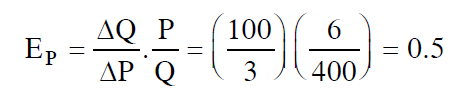If we move from N to M, we get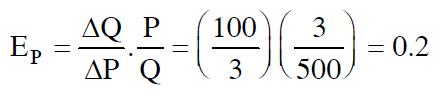Now take the average of the two end values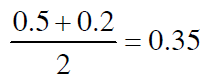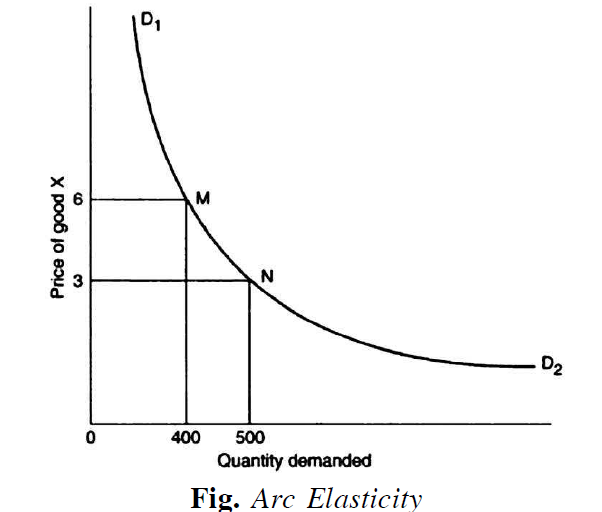Which means it is the price demand that is inelastic.
The arc elasticity is defined as below: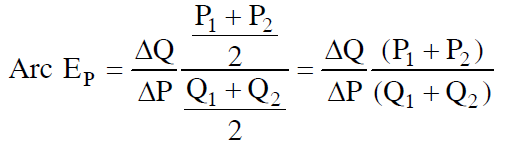Where P1 and P2 are priced before and after changes, Q1 and Q2 are quantities demanded before and after changes respectively. DQ and ΔP refer to change in the quantity demanded and change in the price respectively. To make the arc elasticity more meaningful, compute between the points on the demand curve that is close enough.

• A

Product Possibility Curve• B

Price Factor Curve• C

Expansion Path• D

Product Line• Option : D
• Explanation : The marginal-average rule states that when marginal cost is below average cost, the average cost falls. When marginal cost is above average cost, the average cost rises. When marginal cost equals average cost, the average cost is at its minimum point. Now consider the MC curve in Fig. given below. In the range of output from zero to 6 units per hour, the MC curve is below the AVC curve, and AVC is falling. Beyond 6 units per hour, the MC curve is above AVC, and AVC is rising.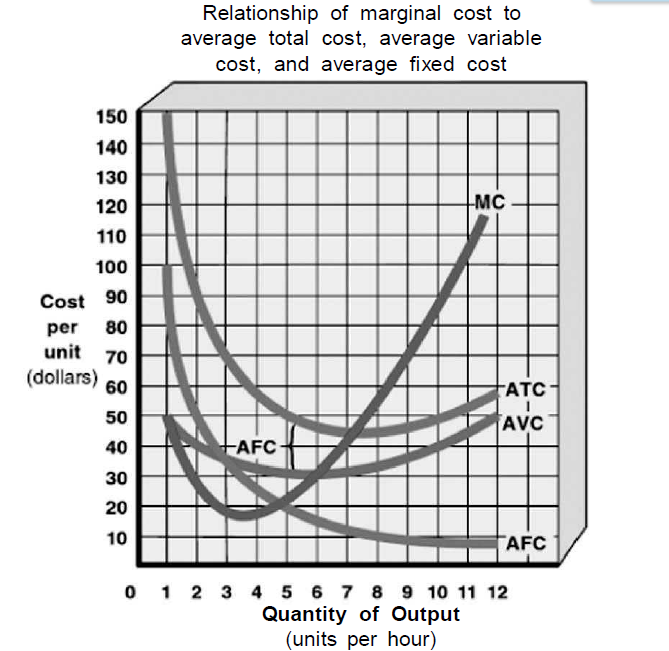• A

Product Tailoring• B

Profit Maximising• C

Monopsony Pricing• D

Public Utility Pricing• Option : B
• Explanation : Inadequacies of Cost-Plus Pricing: > This method ignores demand. The price the consumer is willing to pay is important for purposes of calculating profits. The price the consumer is willing to pay has no relation to costs. Thus, a price based on cost is one-sided.
> This method is easy to operate, but it ignores the nature of competition in the market. Whatever price is fixed is bound to invite reactions from rival firms. But this fact the method ignores. It also ignores the future possibilities of competition as a result of the price policy.
> The cost-plus method assumes that costs can be allocated to individual products. This assumption, as is clear from the example we have taken, is unrealistic. Many costs are common and cannot be traced to individual products.
> It considers full costs. This is not always logical. For planned expansion, incremental costs rather than full costs should be taken as a basis. Also to base future prices on present or past costs is equally illogical.
> Cost-plus pricing suffers from the fallacy of circular reasoning. Sales depend upon price, production depends upon sales, costs depend upon production (because costs change as the level of production change) and price is said to depend upon cost—which completes the circle.